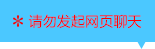| | |

||
APP端下载AndroidiPhone
￥198

|
1961人点赞
616569人已学习
|

3天无理由退款

3天无理由退款：退款将以超级币形式退至您的超级课堂学习账户，便于您重新选购其他课程。恶意退款将被冻结账号。

• 1、并集与交集的性质，主要有$6$组
2、 (1)交换律$A\cup B=B\cup A$，$A\cap B=B\cap A$
3、 (2)结合律$(A\cup B)\cup C=A\cup(B\cup C)$，$(A\cap B)\cap C=A\cap(B\cap C)$
4、 (3)集合与并集、交集的关系$A\subseteq A\cup B$，$B\subseteq A\cup B$；$A\cap B\subseteq A$，$A\cap B\subseteq B$；
5、 (4)集合与本身的并集、交集$A\cup A=A$，$A\cap A=A$，还有推论$A\cup B=A\cap B$，则$A=B$
6、 (5)集合与空集的并集、交集$A\cup\phi =A$，$A\cap\phi =\phi$
7、 (6)并交关系与子集关系的互推，记做“全集并子集=全集，全集交子集=子集”
• 1、一是要把两集合的元素搞清楚，包括元素的性质、元素的个数，在数轴上的范围、或者某些共同特征
2、 二是数轴的运用。记住并集的范围是覆盖的所有区域，交集的范围是覆盖的公共区域。同时考虑并集与交集的范围，就能确定不等式端点的取值范围
3、 三是并集、交集的性质。$A$或$B$中的任意元素，必属于并集。交集中的任意元素，必属于$A$或$B$。根据这两条性质，就能通过交集或并集的元素，探索出$A$、$B$中的元素
• 1、全集和补集的概念，注意：脱离全集去谈补集是没有意义的，而且补集也是全集的子集
2、 补集的两种特殊情形：全集的补集为空集，空集的补集为全集。反之，如果$A$的补集为空集，则$A$为全集；如果$A$的补集为全集，则$A$为空集
3、 补集的图形表示：在数轴或韦恩图上，是全集范围，抠去集合$A$的范围，就是$A$补集的范围。若全集为实数集，求补集就会方便很多，将不等号反过来写就$OK$
• 1、性质一、$A$与$A$的补集都是全集的子集。解题时注意两点：(1)$A$和$A$的补集中不能出现全集中没有的元素；(2)元素的互异性
2、 性质二、集合$A$与$A$补的并集为全集，交集为空集。它告诉我们：全集中的任意一个元素，要么属于$A$，要么属于$A$补，二者必居其一
3、 补集思想的应用：某些抽象、复杂的问题从反面情况思考往往会很简单，尤其是当题目中存在一些特殊词时，比如“至多”、“至少”，就要马上想到“补集思想”，思考问题的反面，情况就会变得简单
• 1、韦恩图由于它的直观，简洁，成为集合解题的必备杀器。一般有两种应用，一种是直接应用，分为两种题型，一种是“由图写集合”，一种“由集合画图”
2、 “由图写集合”是最常见的题型，读懂各种韦恩图的第一步，是要熟悉各种基本的子集、并集、交集、补集还有摩根律、分配率的图形。对于三个或三个以上集合的韦恩图，你要看清阴影部分属于哪些集合，而不属于哪些集合，把它翻译成集合的运算式子。注意“抠图法”，若想抠掉一块区域，可以算跟它补集的交集。
3、 “由集合画图”相对简单很多，照着集合的算式画图就可以确定
• 1、韦恩图的间接应用，引入韦恩图帮助分析集合关系。涉及四类题目：(1)、元素不具体的题目。(2)、自定义的集合运算的题目。(3)、由运算后的集合，反推原集合的题目。(4)、和方程结合求元素个数的题目
2、 第四类题目可以分三步解答： (1)、作图—将题目抽象为集合间的关系，作出韦恩图。(2)、标注—根据条件，通过设未知数，把每个区域的数量都表示出来。(3)、列方程—一般用全集元素的数量，来列方程求解
• 1、对于两个有限集，并集元素个数等于两原集合元素个数相加，再减去交集元素个数。$card(A\cup B)=card(A)+card(B)-card(A\cap B)$
2、 并集元素个数的范围，最小值等于其中元素较多的那个集合的元素个数，发生在两集合有包含关系时。最大值等于两集合元素的个数和，发生在交集为空时。注意$max$这个符号的意思$max\left \{ card(A),card(B) \right \}\leq card(A\cup B)\leq card(A)+card(B)$
3、 对于三个有限集，并集元素个数等于三个原集合元素个数相加，再减去三个两连交集的元素个数，再加上一个三连交集的元素个数。$card(A\cup B)=card(A)+card(B)+card(C)-card(A\cap B)-card(A\cap C)-card(B\cap C)+card(A\cap B\cap C)$
• 1、并集、交集、补集的混合运算，结果依然是集合，运算时从左到右进行，有括号就先算括号内的
2、 对于不等式表示的集合，要借助韦恩图或数轴运算
3、 读懂混合运算，把元素代入集合表达式求参数
4、 混合运算的两大性质，摩根律和分配律
5、 摩根律解释的是两个集合在全集里的交并补关系，分配率解释的则是三个集合的交并关系，它们可以帮我们转化一些复杂的混合运算，让你在集合运算的能力上更胜一筹

• 1rench
• 2• 3超级学员967630
• 4超级学员1105634
• 5超级学员1187098
• 6超级学员1201660
• 7习惯就好y
• 8超级学员1234894
• 9陈情666
• 10超级学员14234331372522人在学
￥ 240 ￥ 0616569人在学
￥ 240 ￥ 1981344780人在学
￥ 240 ￥ 0616613人在学
￥ 300 ￥ 198

• 0
• 点击分享有好礼
••app端下载关注微信号